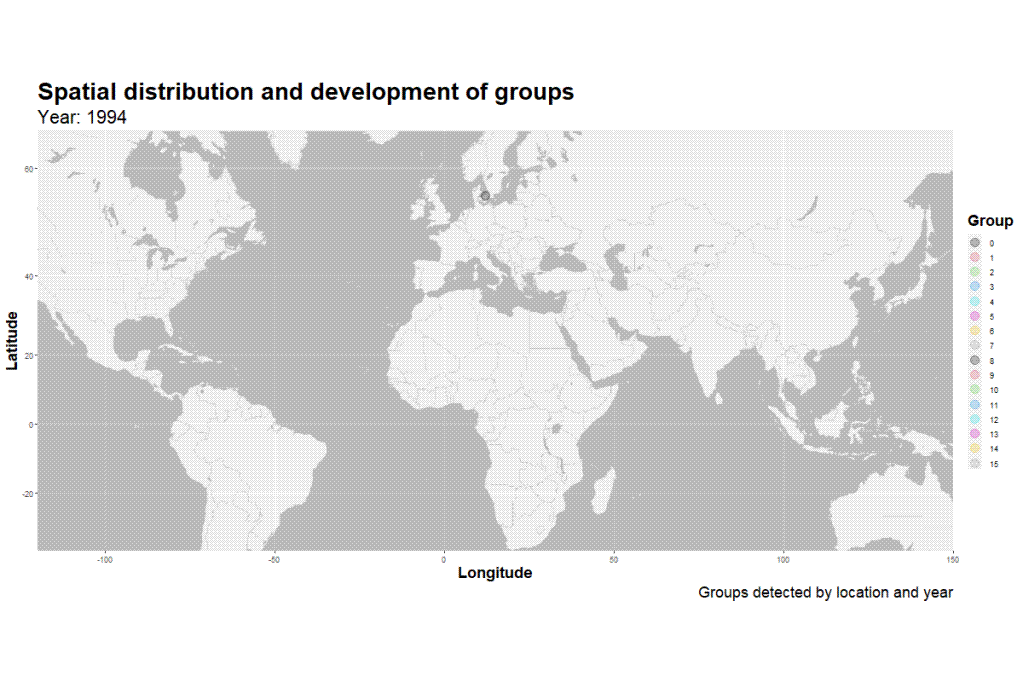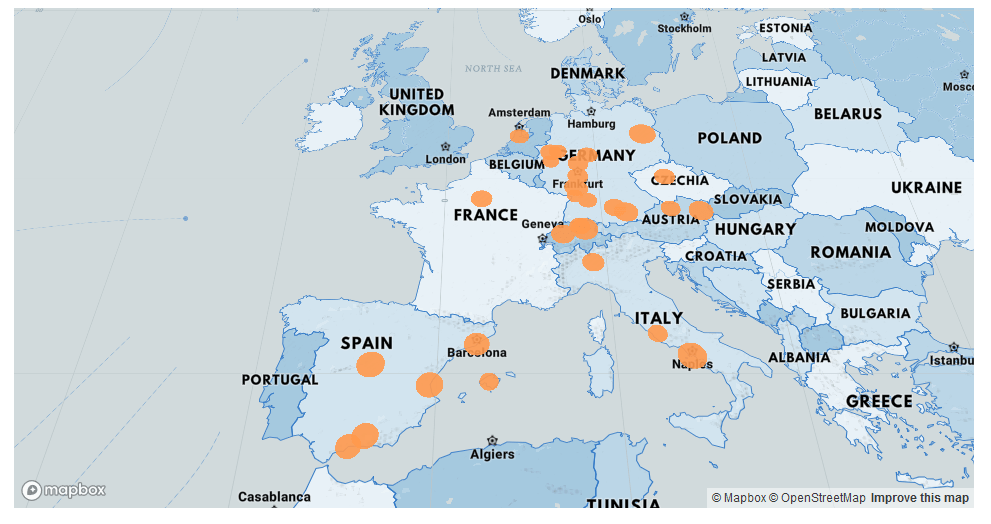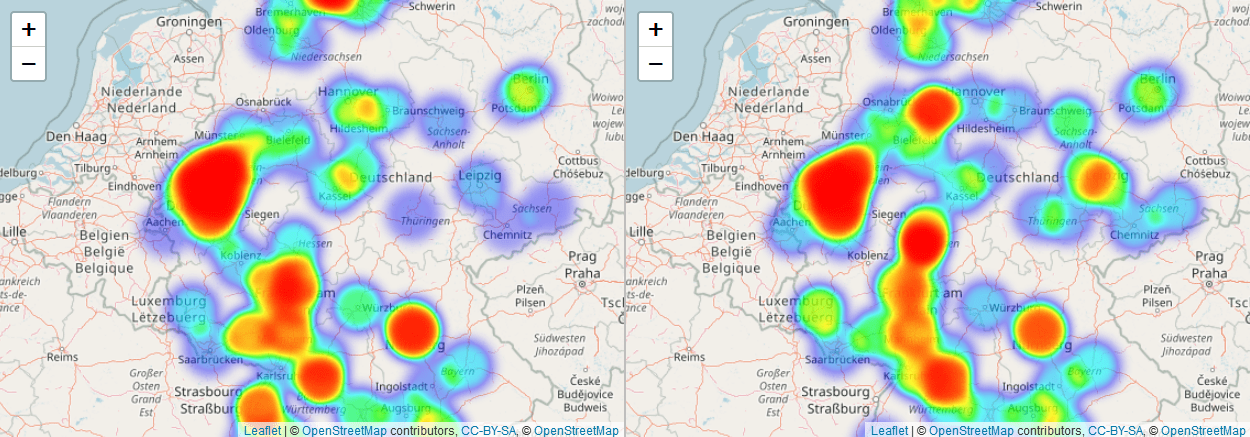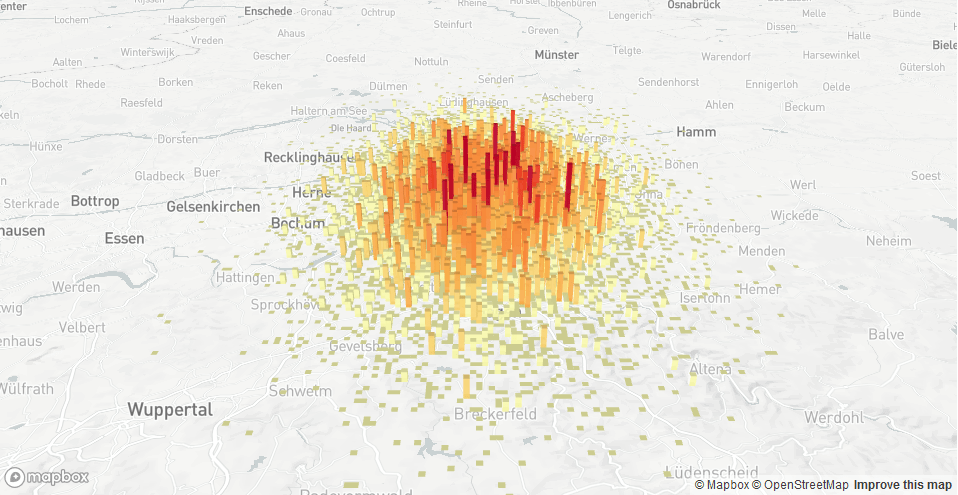# R programming

## Simmer in R for discrete-event simulationThere are various simulation techniques of importance to supply chain data analysis and modelling. One of them is discrete-event simulation. Discrete-event simulation is especially useful for higly dynamic and interdependent systems, as is often the case in complex manufacturing systems […]

## SPSS, SAS and Stata data in R with havenIn this short article I introduce the haven package in R, and how it can be used for importing data from SPSS in SAV-file format into R. I already demonstrated this as part of a longer coding example, in which […]

## Spatial data animation: ggmap & gganimateFollowing a series of introductionary posts on spatial data visualization in R, covering packages such as ggmap, leaflet, tidygeocoder, geopy, deckgl and osmdata, I here present a framework utilizing some relevant packages. The framework was developed in R. It was […]

## Testing tidycovid19 data interface in RThere are various packages available in R for retrieving data on covid19 cases. In a previous post I tested coronavirus, a package available in R providing access to data from J.H. University. In this post I want to quickly introduce […]

## Map-based scatter plots with deckgl in RIn this blogpost I provide a coding example in R for how to create a map-based scatterplot using the deckgl package. This can come in handy when visualising data with some spatial aspect. For example you might want to visualise […]

## Introduction to Leaflet heatmaps in RIn this code example I use a geocoding function found on datascienceplus to geocode Google trends search intensity data, comparing search trend by city name for “Burger” and “Pizza” in Germany. I then visualize the results with heatmaps, generated with […]

## Using deckgl in R for spatial data visualisationI want to provide an example for how to plot 3D map plots with the deckgl package in R. I generate a bar plot on a map layout from Mapbox. First, I define our Open Street Map geocoding function, found […]

## Spatial SCM data visualisation in RIn this post I want to provide a brief overview of useful packages in R for visualizing spatial data in R. The packages discussed are webglobe, deckgl, ggmap, ggplot2 and Leaflet. webglobe The webglobe package allows you to create e.g. […]

## Regression in R: A method comparisonIn this article I provide various examples of regression in R. I compare various regression methods using R. The methods that I will introduce are simple linear regression, multiple linear regression, polynomial regression, decision tree regression, and support vector machine […]